GeeksforGeeks App
Open AppBrowser
Continue

# PySpark – Sort dataframe by multiple columns

In this article, we are going to see how to sort the PySpark dataframe by multiple columns.

It can be done in these ways:

• Using sort()
• Using orderBy()

Creating Dataframe for demonstration:

## Python3

 `# importing module``import` `pyspark`` ` `# importing sparksession from pyspark.sql module``from` `pyspark.sql ``import` `SparkSession`` ` `# creating sparksession and giving an app name``spark ``=` `SparkSession.builder.appName(``'sparkdf'``).getOrCreate()`` ` `# list  of students  data``data ``=` `[[``"1"``, ``"sravan"``, ``"vignan"``],``        ``[``"2"``, ``"ojaswi"``, ``"vvit"``],``        ``[``"3"``, ``"rohith"``, ``"vvit"``],``        ``[``"4"``, ``"sridevi"``, ``"vignan"``],``        ``[``"1"``, ``"sravan"``, ``"vignan"``],``        ``[``"5"``, ``"gnanesh"``, ``"iit"``]]`` ` `# specify column names``columns ``=` `[``'student ID'``, ``'student NAME'``, ``'college'``]`` ` `# creating a dataframe from the lists of data``dataframe ``=` `spark.createDataFrame(data, columns)`` ` `print``(``"Actual data in dataframe"``)`` ` `# show dataframe``dataframe.show()`

Output: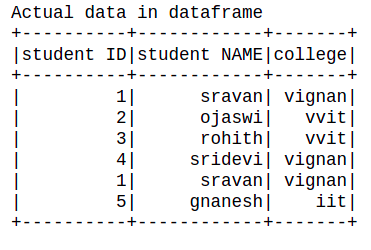### Method 1: Using sort() function

This function is used to sort the column.

Syntax: dataframe.sort([‘column1′,’column2′,’column n’],ascending=True)

Where,

• dataframe is the dataframe name created from the nested lists using pyspark
• where columns are the llst of columns
• ascending = True specifies order the dataframe in increasing order, ascending=False specifies order the dataframe in decreasing order

Example 1: Python code to sort dataframe by passing a list of multiple columns(2 columns) in ascending order.

## Python3

 `# show dataframe by sorting the dataframe``# based on two columns in ascending order``dataframe.sort([``'college'``,``'student ID'``],``               ``ascending ``=` `True``).show()`

Output: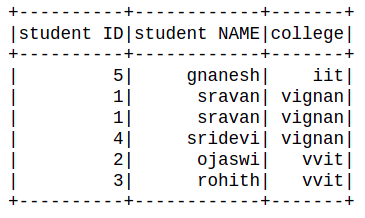Example 2: Python program to sort the data frame by passing a list of columns in descending order

## Python3

 `# show dataframe by sorting the dataframe``# based on two columns in descending order``dataframe.sort([``'college'``,``'student NAME'``],``               ``ascending ``=` `False``).show()`

Output: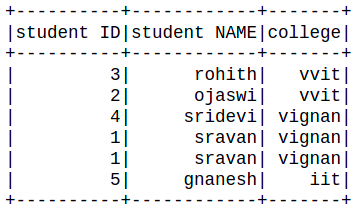### Method 2: Using orderBy() function.

orderBy() function that sorts one or more columns. By default, it orders by ascending.

Syntax: orderBy(*cols, ascending=True)

Parameters:

• cols: Columns by which sorting is needed to be performed.
• ascending: Boolean value to say that sorting is to be done in ascending order

Example 1: Python program to show dataframe by sorting the dataframe based on two columns in descending order using orderby() function

## Python3

 `# show dataframe by sorting the dataframe``# based on two columns in descending``# order using orderby() function``dataframe.orderBy([``'student ID'``,``'student NAME'``],``                  ``ascending ``=` `False``).show()`

Output: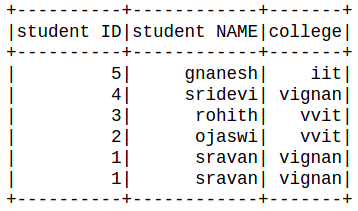Example 2: Python program to show dataframe by sorting the dataframe based on two columns in ascending order using orderby() function

## Python3

 `# show dataframe by sorting the dataframe``# based on two columns in ascending``# order using orderby() function``dataframe.orderBy([``'student ID'``,``'student NAME'``],``                  ``ascending ``=` `True``).show()`

Output: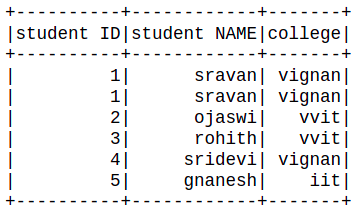My Personal Notes arrow_drop_up